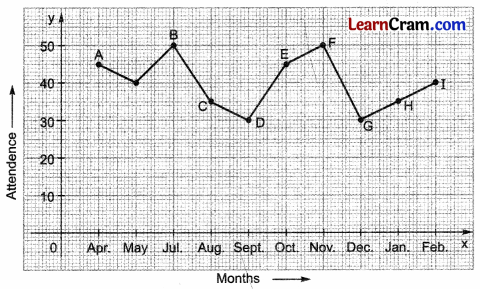# DAV Class 8 Maths Chapter 13 Brain Teasers Solutions

The DAV Maths Book Class 8 Solutions Pdf and DAV Class 8 Maths Chapter 13 Brain Teasers Solutions of Introduction to Graphs offer comprehensive answers to textbook questions.

## DAV Class 8 Maths Ch 13 Brain Teasers Solutions

Question 1A.
Tick (✓) the correct option.
(i) The point at which the x-axis and y-axis intersect on the cartesian plane is
(a) abscissa
(b) origin
(c) ordinate
(d) none
Solution:
The x-axis and y-axis intersect each other on the cartesian plane at the origin. Thus, option (b) is the correct answer.

(ii) If a point lies on the y-axis, its coordinates are
(a) (x, 0)
(b) (0, 0)
(c) (x, y)
(d) (0, y)
Solution:
If a point lies on the y-axis, its abscissa is 0 and the ordinate is y. Hence, its coordinates are (0, y)Thus, option (d) is the correct answer.(iii) The distance of a point P(2, 3) from x-axis is
(a) 3 units
(b) 2 units
(c) 5 units
(d) 1 unit
Solution:
The ordinate represents the distance from the x-axis of a point. Here, the ordinate of P (2, 3) is 3. So the given point is 3 units away from the x-axis. Thus, option (a) is the correct answer.

(iv) The distance of a point from the y-axis is called
(a) ordinate
(b) y-coordinate
(c) abscissa
(d) none
Solution:
The abscissa represents the distance from the y-axis of a point. Thus, option (c) is the correct answer.

Question 1B.
(i) Write down the coordinates of the origin.
Solution:
The coordinates of the origin are (0, 0).

(ii) What are the coordinates of a point which lies on the x-axis?
Solution:
The coordinates of a point that lies on the x-axis are (x, 0).

(iii) What is the distance of point C(4, 2) from the y-axis?
Solution:
Abscissa represents the distance of a point from the y-axis. So, the distance of point C(4, 2) is 4 units.

(iv) What is the distance between the points P (0, 2) and Q (0, 6)?Solution:
Both Points P(0, 2) and Q(0, 6) lie on the y-axis as the abscissa of both is 0.
∴ The distance between both the points = Difference of ordinates
= 6 – 2
= 4 units

(v) If the vertices O, A, B of a square OABC are (0, 0), (2, 0), and (2, 2), what are the coordinates of the fourth vertex C of the square?
Solution: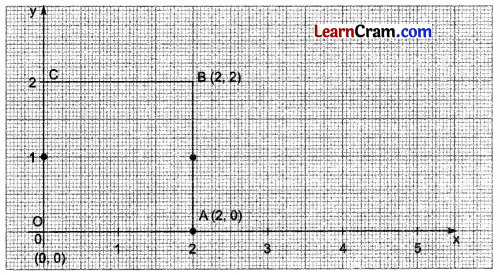Plot points O(0, 0), A(2, 0) and B(2, 2) on the graph. Join A to B and B to Y-axis at point C, Point C is the fourth vertex of the square ABCD. Coordinates of point C are (0, 2).Question 2.
Write the coordinates of each of the vertices of each polygon given below: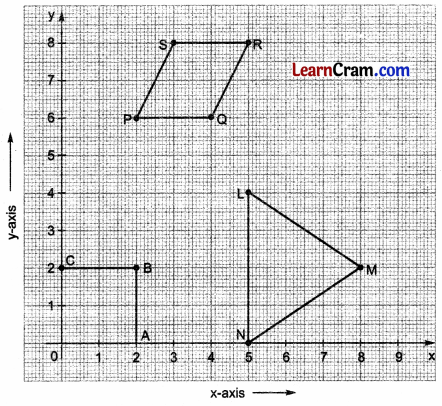Solution:
(i) A(2, 0)
(ii) B(2, 2)
(iii) C(o, 2)
(iv) N(5, 0)
(v) L(5, 4)
(vi) M(8, 2)
(vii) P(2, 6)
(viii) Q(4, 6)
(ix) R(5, 8)
(x) S(3, 8)

Question 3.
The runs scored by the Indian and Australian teams in first 10 overs are given below:

 Overs I II III IV V VI VII VIII IX X India Runs 3 4 6 5 7 8 10 5 6 4 Australia Runs 5 6 8 9 10 12 4 6 8 8

Draw a graph showing the data making the graphs on the same axes in each case.
Solution:
Scale on x-axis: each over = 1 cm
Scale on the y-axis: 1 run = 1 cm### DAV Class 8 Maths Chapter 13 HOTS

Question 1.
On a cartesian plane, plot the given points A(1, 1), B(5, 1), C(5, 5) and D(1, 5). Join the points in order.
(i) What figure do you get?
(ii) White coordinates of the point of intersection of the diagonals.
(iii) Are the diagonals equal? Do they intersect each other at right angles? (Use scale and protractor)
Solution:
(i) By plotting the given points and joining them in order, we get a square (see graph).
(ii) Diagonals of square ABCD intersect each other at O. Coordinates of point O are (3, 3).
(iii) Yes, the diagonals of the square are equal and they interact with each other at a right angle.
Scale on the x-axis: 1 cm = 1 unit
Scale on the y-axis: 1 cm = 1 unitQuestion 2.
On a cartesian plane, draw a square whose point of intersection of the diagonals is (8, 3) and one vertex is (6, 1).
Solution:
Scale on the x-axis: 1 cm = 1 unit
Scale on the y-axis: 1 cm = 1 unit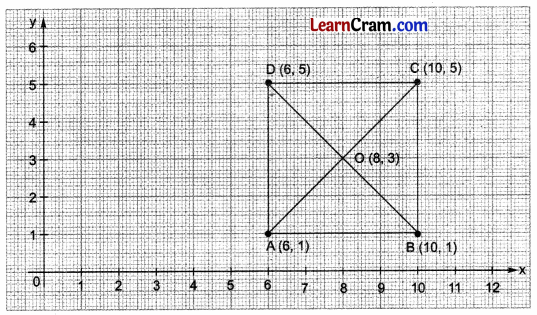### DAV Class 8 Maths Chapter 13 Enrichment Questions

Question 1.
On a cartesian plane, draw a rectangle whose two vertices are (2, 1) and (6, 6). Write the coordinates of the other two vertices.
Solution:
ABCD is the required rectangle whose vertices are A(2, 1), B(6, 1), C(6, 6) and D(2, 6). Thus, the coordinates of the other two vertices are (6, 1) and (2, 6).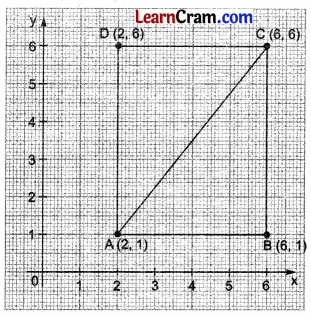Question 2.
On a cartesian plane, draw a line segment XY parallel to the x-axis at a distance of 5 units from the x-axis and a line segment PQ parallel to the y-axis at a distance of 3 units from the y-axis. Write the coordinates of their point of intersection.
Solution:O is the point of intersection of lines XY and PQ.
The coordinates of point O are (3, 5).

Question 1.
The number of students in a hostel, speaking different languages is given below. Represent the information in a graph.

 Language Hindi English Marathi Tamil Bengali Number of Students 40 12 9 7 4

Solution:Question 2.
In the given figure, indicate the coordinates of the vertices of the polygon ABODE.Solution:
Coordinates of the vertices of the polygon ABODE are given as:
(i) A(1, 5)
(ii) B(3, 8)
(iii) C(6, 7)
(iv) D(5, 3)
(v) E(3, 2)

Question 3.
The number of shirts sold by a retailer in a particular week is given below:

 Days 1 2 3 4 5 6 7 No.of Shirts Sold 25 30 40 25 15 10 50

Represent the above information in a graph.
Solution:
Scale on x-axis: 1 day = 1 cm
Scale on the y-axis: 10 shirts = 1 cm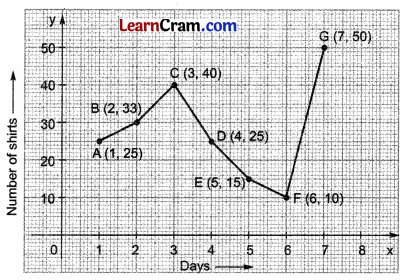Question 4.
The pulse rates of a patient at different times during the day are given below. Draw the pulse rate-time graph.

 Time in Hours 6:00 8:00 10:00 12:00 14:00 16:00 Pulse Rate 70 60 80 90 45 75

Solution:
Scale on x-axis: 1 hr = 1 cm
Scale on the y-axis: 10 shirts = 1 cmQuestion 5.
The following table shows the attendance of students in each month of 2012-2013 for a class of 50 students. Represent the information in the graph:

 Months April May July Aug. Sept. Oct. Nov. Dec. Jan. Feb. Attendance 45 40 50 35 30 45 50 30 35 40

Solution:
Scale on x-axis: 1 month = 1 cm
Scale on the y-axis: 10 students = 1 cm